# How to use superscript(power) in LaTeX?

Superscripts are very commonly used in mathematical expressions as exponents and in some special operators. In this tutorial, we will discuss how to use Superscript in LaTeX.

## Superscript in math mode

To use Superscript with a symbol or character in LaTeX, you need to use the ^ key on the keyboard.

For example, if you want to print , you have to use x^2 and you must write it in Math mode of LaTeX. Below is an example using superscript with some symbols.

\documentclass{article}
\usepackage{amssymb}
\begin{document}
$\verb|x^n|\dashrightarrow x^n$
$\verb|\sum^m|\dashrightarrow \sum^m$
$\verb|\prod^n|\dashrightarrow \prod^n$
$\verb|\cup^n|\dashrightarrow \cup^n$
$\verb|\sigma^n|\dashrightarrow \sigma^n$
\end{document}

Output :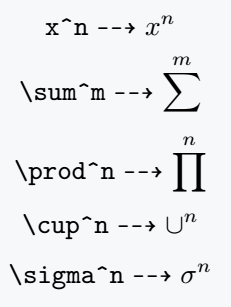If you want to use more than one character in Superscript, you need to put the entire Superscript part in curly brackets {..}, otherwise only the 1st character will be rendered in the superscript position. Take a look.

\documentclass{article}
\usepackage{amssymb}
\begin{document}
$\verb|x^mn|\dashrightarrow x^mn \quad \verb|x^{mn}|\dashrightarrow x^{mn}$
$\verb|x^{a+b}|\dashrightarrow x^{(a+b)}$
$\verb|x^{\frac{\pi}{2}}|\dashrightarrow x^{\frac{\pi}{2}}$
\end{document}

Output :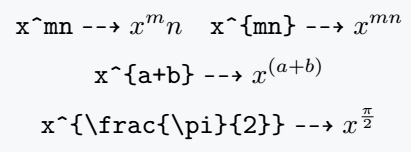If you want to place the position of the exponent a little higher then you have to write the command like this x^{\!^{exponent}}.

\documentclass{article}
\usepackage{amssymb}
\begin{document}
$\verb|\alpha^{\!^{n}}|\dashrightarrow \alpha^{\!^{n}}$
$\verb|x^{\!^{\frac{\pi}{2}}}|\dashrightarrow x^{\!^{\frac{\pi}{2}}}$
$\verb|x^{\!^{\left(\frac{a+b}{2}\right)}}|\dashrightarrow x^{\!^{\left(\frac{a+b}{2}\right)}}$
\end{document}

Output :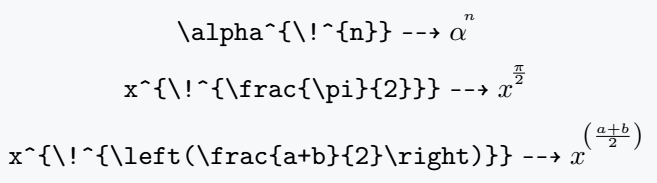## Superscript in text mode

To use superscript outside math, LaTeX provides a command called \textsuperscript{}.

With this command you can easily use superscript in text mode and this command can also be used in math mode but I would not recommend using this command in math mode.

\documentclass{article}
\usepackage{amssymb}
\begin{document}
\verb|n\textsuperscript{th}|$\dashrightarrow$ n\textsuperscript{th}\$4pt] Finding n\textsuperscript{th} number made of prime digits.\\[4pt] 1\textsuperscript{st}\quad2\textsuperscript{nd}\quad3\textsuperscript{rd} \end{document} Output :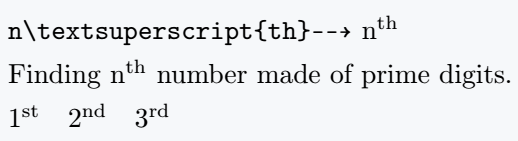## Multiple superscripts in LaTeX Many times we need multiple superscripts. But, many of you will be wrong in defining. The following code will help you to represent it properly. \documentclass{article} \begin{document} \[ (x^a)^b =(x^b)^a =x^{ab}$
$x^{abc}\quad x^{pqr}$
$x^{a^{b^c}}\quad x^{p^{q^r}}$
\end{document}

Output :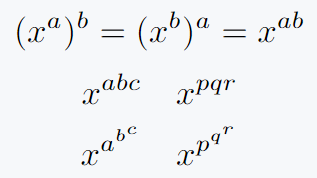Inverse diagonal dots will be needed to represent this continuous superscript. For this, the adots command of the yhmath package has been used.

Curly brackets are also very important to properly represent this expression. Of course, you can easily understand by looking at the code below.

\documentclass{article}
\usepackage{yhmath}
\begin{document}
$\sum_{i=0}^n x_n = x^{x_1^{x_2^{x_3^{\adots^{x_n}}}}}$
$\exp \left(e^{e^{\adots^\infty}} \right)= e^{e^{e^{\adots^\infty}}}$
\end{document}

Output :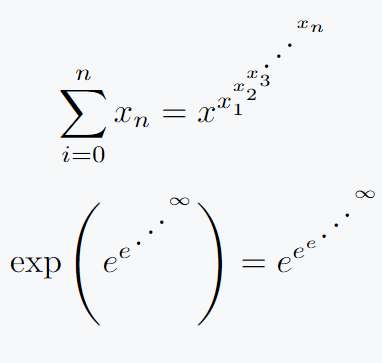#### Md Jidan Mondal

LaTeX expert with over 10 years of experience in document preparation and typesetting. Specializes in creating professional documents, reports, and presentations using LaTeX.